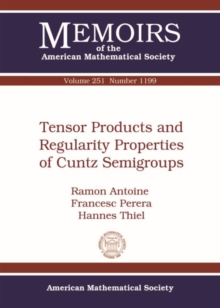Supporting your high street Find out how »
• My Account# Tensor Products and Regularity Properties of Cuntz Semigroups Paperback / softback

## Part of the Memoirs of the American Mathematical Society series

#### Description

The Cuntz semigroup of a $C^*$-algebra is an important invariant in the structure and classification theory of $C^*$-algebras.

It captures more information than $K$-theory but is often more delicate to handle.

The authors systematically study the lattice and category theoretic aspects of Cuntz semigroups. Given a $C^*$-algebra $A$, its (concrete) Cuntz semigroup $\mathrm{Cu}(A)$ is an object in the category $\mathrm{Cu}$ of (abstract) Cuntz semigroups, as introduced by Coward, Elliott and Ivanescu.

To clarify the distinction between concrete and abstract Cuntz semigroups, the authors call the latter $\mathrm{Cu}$-semigroups. The authors establish the existence of tensor products in the category $\mathrm{Cu}$ and study the basic properties of this construction.

They show that $\mathrm{Cu}$ is a symmetric, monoidal category and relate $\mathrm{Cu}(A\otimes B)$ with $\mathrm{Cu}(A)\otimes_{\mathrm{Cu}}\mathrm{Cu}(B)$ for certain classes of $C^*$-algebras. As a main tool for their approach the authors introduce the category $\mathrm{W}$ of pre-completed Cuntz semigroups.

They show that $\mathrm{Cu}$ is a full, reflective subcategory of $\mathrm{W}$.

One can then easily deduce properties of $\mathrm{Cu}$ from respective properties of $\mathrm{W}$, for example the existence of tensor products and inductive limits.

The advantage is that constructions in $\mathrm{W}$ are much easier since the objects are purely algebraic.

#### Information

• Format: Paperback / softback
• Pages: 191 pages
• Publisher: American Mathematical Society
• Publication Date:
• Category: Algebra
• ISBN: 9781470427979

£77.95

£77.55£84.50

£82.85

£84.50

£83.89

£84.50

£83.89

£84.50

£83.89